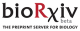Title Topological and Kinetic Determinants of the Modal Matrices of Dynamic Models of Metabolism Year of Publication 2017 Authors B. Du; D.C. Zielinski; B.O. Palsson Journal PLoS Comput Biol Abstract Linear analysis of kinetic models of metabolism can help in understanding the dynamic response of metabolic networks. Central to linear analysis of these models are two key matrices: the Jacobian matrix (J) and its modal matrix (M-1). The modal matrix contains dynamically independent motions of the kinetic model, and it is sparse in practice. Understanding the sparsity structure of the modal matrix provides insight into metabolic network dynamics. In this study, we analyze the relationship between J and M-1. First, we show that diagonal dominance occurs in a substantial fraction of the rows of J, resulting in simple modal structures within M-1. Dominant diagonal elements in J approximate the eigenvalues corresponding to these simple modes, in which a single metabolite is driven back to its reference state on a characteristic timescale. Second, we analyze more complicated mode structures in M-1, in which two or more variables move in a certain ratio relative to one another on defined time scales. We show that complicated modes originate from sub-matrices of topologically connected elements of similar magnitude in J. Third, we describe the origin of these mode structure features based on the network stoichiometric matrix S and the reaction kinetic gradient matrix G. We demonstrate that the topologically-connected reaction sensitivities of similar magnitude in G play a central role in determining the mode structure. Ratios of these reaction sensitivities represent equilibrium balances of half reactions that are defined by linearization of the bilinear mass action rate laws followed by enzymatic reactions. These half-reaction equilibrium ratios are key determinants of modal structure for both simple and complicated modes. The work presented here helps to establish a foundation for understanding the dynamics of kinetic models of metabolism, which are rooted in the network structure and the kinetic properties of reactions.     URL https://doi.org/10.1101/107425